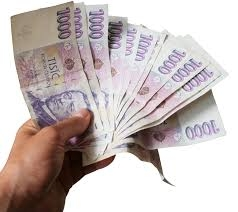# Banknotes 17573

The eldest sister saved CZK 7,800 in the bank. She brought this amount only in 100CZK and 200CZK banknotes. The total number of these banknotes was 50. How many banknotes of both values ​​were there?

a =  22
b =  28

### Step-by-step explanation:

7800 = 100a + 200b
a+b=50

7800 = 100·a + 200·b
a+b=50

100a+200b = 7800
a+b = 50

Row 2 - 1/100 · Row 1 → Row 2
100a+200b = 7800
-b = -28

b = -28/-1 = 28
a = 7800-200b/100 = 7800-200 · 28/100 = 22

a = 22
b = 28

Our linear equations calculator calculates it.Did you find an error or inaccuracy? Feel free to write us. Thank you!

Tips for related online calculators
Do you have a linear equation or system of equations and looking for its solution? Or do you have a quadratic equation?